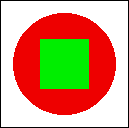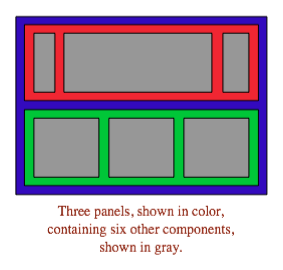[ Quiz Answers | Chapter Index | Main Index ]

## Quiz on Chapter 6

This page contains questions on Chapter 6 of Introduction to Programming Using Java. You should be able to answer these questions about studying that chapter. Sample answers to these questions can be found here.

 Question 1: Programs written for a graphical user interface have to deal with "events." Explain what is meant by the term event. Give at least two different examples of events, and discuss how a program might respond to those events. Question 2: Explain carefully what the repaint() method does. Question 3: What is HTML? Question 4: Java has a standard class called JPanel. Discuss two ways in which JPanels can be used. Question 5: Draw the picture that will be produced by the following paintComponent() method: ```public static void paintComponent(Graphics g) { super.paintComponent(g); for (int i=10; i <= 210; i = i + 50) for (int j = 10; j <= 210; j = j + 50) g.drawLine(i,10,j,60); }``` Question 6: Suppose you would like a panel that displays a green square inside a red circle, as illustrated. Write a paintComponent() method for the panel class that will draw the image.Question 7: Java has a standard class called MouseEvent. What is the purpose of this class? What does an object of type MouseEvent do? Question 8: One of the main classes in Swing is the JComponent class. What is meant by a component? What are some examples? Question 9: What is the function of a LayoutManager in Java? Question 10: What type of layout manager is being used for each of the three panels in the following illustration from Section 6.7?Question 11: Explain how Timers are used to do animation. Question 12: What is a JCheckBox and how is it used?

[ Quiz Answers | Chapter Index | Main Index ]